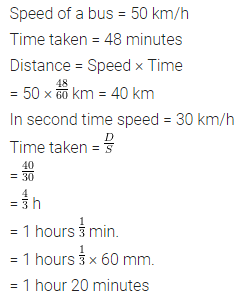ML Aggarwal Class 7 Solutions Chapter 6 Ratio and Proportion Ex 6.4 for ICSE Understanding Mathematics acts as the best resource during your learning and helps you score well in your exams.

## ML Aggarwal Class 7 Solutions for ICSE Maths Chapter 6 Ratio and Proportion Ex 6.4

Question 1.
Convert the following speeds into m/sec:
(i) 72 km/h
(ii) 9 km/h
(iii) 1.2 km/minutes
(iv) 600 m/hour
Solution: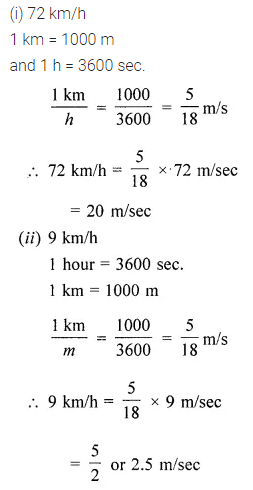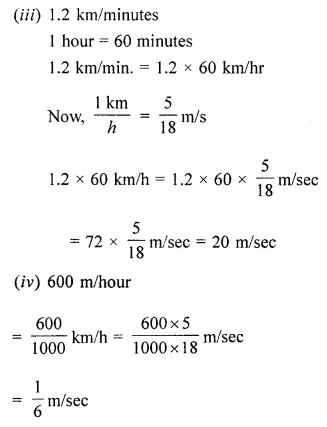Question 2.
Convert the following speeds into km/h:
(i) 15 m/sec
(ii) 1.5 m/sec
Solution: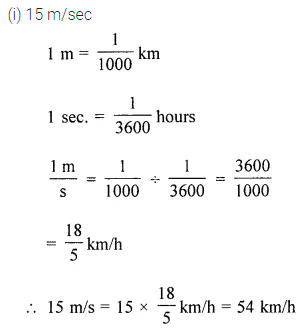Question 3.
Which is greater- the speed of 30 m/sec or 30 km/h?
Solution:Question 4.
An aeroplane is flying at a speed of 720 km/h.
(i) If the aerial distance between two cities is 1800 km, how much time will the aeroplane take in crossing these cities?
(ii) How much distance does the aeroplane cover in 40 minutes?
(iii) How far will it fly in 15 seconds?
Solution: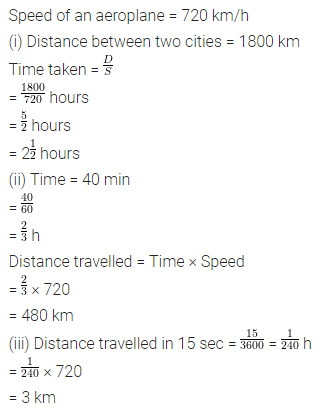Question 5.
A dog is walking at a speed of 6 km/h.
(i) How much distance does it cover in 5 minutes?
(ii) How much time would it take to cover 200 metres?
Solution: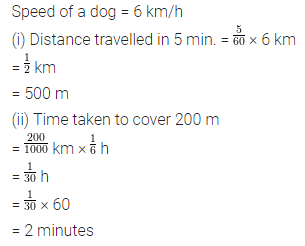Question 6.
A swimming pool is 50 metres long. A boy can swim across the length and then return to his starting position in 5 minutes. What is his swimming speed in km/h?
Solution: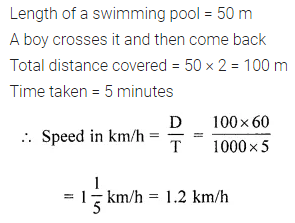Question 7.
A bus takes 48 minutes to cover a certain distance when travelling at a speed of 50 km/h. How much time will it take to cover the same distance when travelling at a speed of 30 km/h?
Solution: